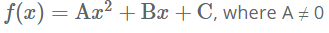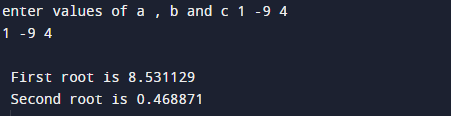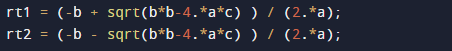C Program to Solve Second Order Quadratic Equation

In this tutorial you will learn about the C Program to Solve Second Order Quadratic Equation and its application with practical example.

In this tutorial, we will learn to create a C program that will find Second Order Quadratic Equation and a C program of it using math.h header file using C programming.

Prerequisites

Before starting with this tutorial we assume that you are best aware of the following C programming topics:

• C Operators
• Looping statements
• Basic input/output.
• Basic C Programming
• Inbuilt library functions.

What Is Second Order Quadratic Equation?

In Algebra, a quadratic equation is, a equation whose quadratic polynomial or, a polynomial of degree 2 is a function with 1 or more variables in which the highest-degree term is of the second degree.Second degree polynomial, also referred as a quadratic equation .Polynomial function whose general form is:=>C Program to Solve Second Order Quadratic Equation

In this program we will find quadratic equation.First of all we declare and initialized the required variables.
Next,we would prompt user to input the values of a,b and c then with the help of sqrt() function we will calculate the first and second root or equations.

OutputIn the above program, we have first declared and initialized a set variables required in the program.

• a,b,c = for holding coordinates
• rt1= shows first root.
• rt2= shows second root.

In this program first of we prompted user to input values of a,b and c after getting the values of a,b and c and with the help of inbuilt function sqrt.whose define in math.h header file we going to find the Second Order Quadratic Equation.as we know the formula of Quadratic Equation. we will use formula to get first and second root of given coordinates using c program.

In this tutorial we have learn about the C Program to Solve Second Order Quadratic Equation and its application with practical example. I hope you will like this tutorial.